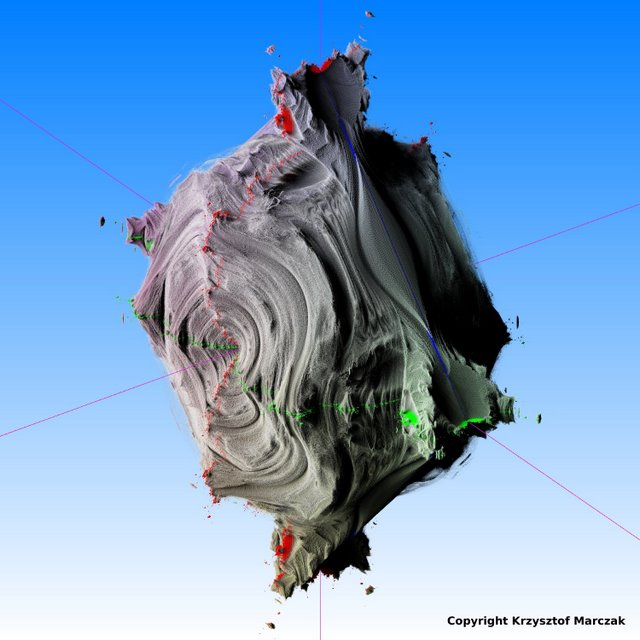News: Support us via Flattr FLATTR Link## The All New FractalForums is now in Public Beta Testing! Visit FractalForums.org and check it out!

 Pages:    Go DownAuthor Topic: 3D Mandelbrot type z^3 + p  (Read 4010 times) Description: 0 Members and 1 Guest are viewing this topic.
Buddhi
Fractal IambusPosts: 895« on: July 19, 2009, 09:40:28 AM »

Hi
I have just render my first 3D Mandelbrot with z in 3-rd power:

z(n+1) = z(n)^3 + p

where z is Quatermion:
z = ra + ib + jc + kd

multiplying rules:
r*i = i; r*j = j; r*k=k;
r*r=r; i*i=-1; j*j=-1; k*k=-1;
i*j = k; j*k=i; k*i=j;

I used 4D Quaternions to make 4D formula for render 3D fractal on 2D screen :-). After very long multiplying on paper (two A4 pages :-) I have got following formula for iterations:
Code:
newx = x*x*x - 3*(x*y*y + 3*x*z*z + 3*x*w*w) - 6*y*z*w + a
newy = -y*y*y + 3*(x*x*y - y*z*z - y*w*w) + 6*z*z*w + b
newz = -z*z*z + 3*(x*x*z - z*y*y - z*w*w) + 6*x*y*w + c
neww = -w*w*w + 3*(x*x*w - w*y*y - w*z*z) + 6*x*y*z + d   (d = 0)http://www.fractalforums.com/gallery/0/640_19_07_09_9_36_29.jpgLogged

David Makin
Global Moderator
Fractal SeniorPosts: 2286« Reply #1 on: July 19, 2009, 02:46:17 PM »

Hi
I have just render my first 3D Mandelbrot with z in 3-rd power:

z(n+1) = z(n)^3 + p

where z is Quatermion:
z = ra + ib + jc + kd

multiplying rules:
r*i = i; r*j = j; r*k=k;
r*r=r; i*i=-1; j*j=-1; k*k=-1;
i*j = k; j*k=i; k*i=j;

I used 4D Quaternions to make 4D formula for render 3D fractal on 2D screen :-). After very long multiplying on paper (two A4 pages :-) I have got following formula for iterations:
Code:
newx = x*x*x - 3*(x*y*y + 3*x*z*z + 3*x*w*w) - 6*y*z*w + a
newy = -y*y*y + 3*(x*x*y - y*z*z - y*w*w) + 6*z*z*w + b
newz = -z*z*z + 3*(x*x*z - z*y*y - z*w*w) + 6*x*y*w + c
neww = -w*w*w + 3*(x*x*w - w*y*y - w*z*z) + 6*x*y*z + d   (d = 0)

Nice to see that it seems to "work" at higher powers tooJust a small point - you shouldn't really call it quaternionic because quaternions are a very specific 4D number form not a general term for 4D number systems and this system is quite different from the quaternionsLogged

The meaning and purpose of life is to give life purpose and meaning.

http://www.fractalgallery.co.uk/
"Makin' Magic Music" on Jango
Buddhi
Fractal IambusPosts: 895« Reply #2 on: July 19, 2009, 03:02:51 PM »

Just a small point - you shouldn't really call it quaternionic because quaternions are a very specific 4D number form not a general term for 4D number systems and this system is quite different from the quaternionsI agree with you. I know that multiplication of quaternions is not commutative and in our calculations we use commutative multiplication (ore some custom multiplication matrix). We use number system only SIMILAR to quaternions. But it WORKS!Logged

cKleinhuis
Fractal SeniorPosts: 7044formerly known as 'Trifox'« Reply #3 on: July 20, 2009, 02:43:34 AM »

this seems to be working really ...i can see the shape of the z^3 shape vertically and horizontally, nice work !note: could it be that the shape is non-fractal around  the 45 deegree ? this might be the visual outcome of the drawback used formulas....

 « Last Edit: July 20, 2009, 03:04:23 AM by Trifox »Logged

---

divide and conquer - iterate and rule - chaos is No random!
lycium
Fractal SupremoPosts: 1158« Reply #4 on: July 20, 2009, 04:17:50 AM »

Just a small point - you shouldn't really call it quaternionic because quaternions are a very specific 4D number form not a general term for 4D number systems and this system is quite different from the quaternionsI agree with you. I know that multiplication of quaternions is not commutative and in our calculations we use commutative multiplication (ore some custom multiplication matrix). We use number system only SIMILAR to quaternions. But it WORKS!

by that reasoning we could call cars "dogs" instead because they both have "four thingies on the ground"Logged

 Pages:    Go Down
 Related Topics Subject Started by Replies Views Last posttype e (new type.. modified xyz vs mag formula) Images Showcase (Rate My Fractal) M Benesi 0 2508May 19, 2010, 09:51:09 AM by M BenesiChaosbrot type c2 improved (new type again, awesome variety) The 3D Mandelbulb M Benesi 1 3960June 10, 2010, 09:24:42 AM by M BenesiNew fractal type... latest 3d type.. a z^2 for Benoit Images Showcase (Rate My Fractal) M Benesi 0 5641October 21, 2010, 07:14:00 AM by M BenesiAnother (?) 3D mandelbrot type Theory Tater 0 1598January 28, 2013, 10:47:15 PM by TaterNew type of 3D Mandelbrot never before seen?? 3D Fractal Generation realflow100 10 2549May 29, 2017, 01:52:09 AM by SamTiba